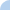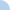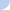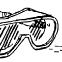# Multiplication Short Cuts # 1 Multiplying by 25

Multiplying by 25

Step 1: divide the number by 4
Step 2: multiply that number by 100

When dividing by 4, if there is a remainder follow the guidelines below:
remainder of 1 ends in 25
remainder of 2 ends in 50
remainder of 3 ends in 75
remainder of 0 ends in 00First name Last nameMrs. Seman 7th Grade Science Instructor Kirby World Academy Wichita Falls, TX